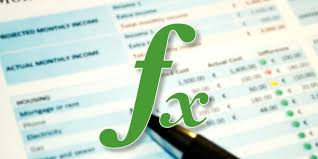### Excel Functions & Formulas ebook

Excel Functions tutorialDiscover how functions in Excel help you save time. If you are new to functions in Excel, we recommend you to read our introduction to Formulas and Functions first.

# Contents:

Count and Sum: The most used functions in Excel are the functions that count and sum. You can count and sum based on one criteria or multiple criteria.

Logical: Learn how to use Excel'slogical functions such as the IF, AND and OR function.

Cell References: Cell references in Excel are very important. Understand the difference between relative, absolute and mixed reference, and you are on your way to success.

Date & Time: To enter a date in Excel, use the "/" or "-" characters. To enter a time, use the ":" (colon). You can also enter a date and a time in one cell.

Text: Excel has many functions to offer when it comes to manipulating text strings.

Lookup & Reference: Learn all about Excel's lookup & reference functions such as the VLOOKUP, HLOOKUP, MATCH, INDEX and CHOOSE function.

Financial: This chapter illustrates Excel's most popular financial functions.

Statistical: An overview of some very useful statistical functions in Excel.

Round:
This chapter illustrates three functions to round numbers in Excel. The ROUND, ROUNDUP and ROUNDDOWN function.

Formula Errors: This chapter teaches you how to deal with some common formula errors in Excel.

Array Formulas: This chapter helps you understand array formulas in Excel. Single cell array formulas perform multiple calculations in one cell.